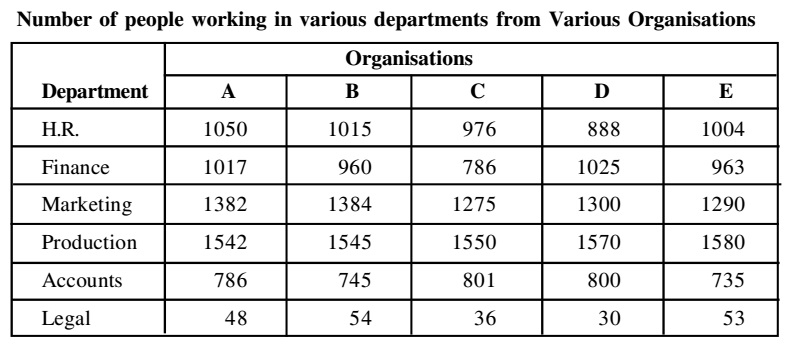## Table chart

#### Data Interpretation

Direction: Study the following table carefully and answer the questions given below :1. What is the total number of employees from all the departments working in all the organisations together?

1. Number of employees working in different organisation are
A → 1050 + 1017 + 1382 + 1542 + 786 + 48 = 5825
Similarly calculate the number of employee from other organisation from given table graph.

##### Correct Option: B

Number of employees working in different organisation are
A → 1050 + 1017 + 1382 + 1542 + 786 + 48 = 5825
B → 1015 + 960 + 1384 + 1545 + 745 + 54 = 5703
C → 976 + 786 + 1275 + 1550 + 801 + 36 = 5424
D → 888 + 1025 + 1300 + 1570 +800 + 30 = 5613
E → 1004 + 963 + 1290 + 1580 + 735 + 53 = 5625
Hence, total number of employees = 5825 + 5703 + 5424 + 5613 + 5625 = 28190

1. What is the approximate difference between the average number of people working in Marketing and production departments from all the organisations together?

1. Total number of employees working in Marketing department = 1382 + 1384 + 1275 + 1300 + 1290 = 6631
Total number of employees working in production department = 1542 + 1545 + 1550 + 1570 + 1580 = 7787

##### Correct Option: A

Total number of employees working in Marketing department = 1382 + 1384 + 1275 + 1300 + 1290 = 6631
Total number of employees working in production department = 1542 + 1545 + 1550 + 1570 + 1580 = 7787
Their difference = 77 - 6631 = 1156
Hence, average difference = 1156/5 = 231.2 ≈ 231

1. The total number of employees working in the Legal Department are approximately what percent of the total number of employees working in H.R. Department of all the organisations together?

1. Total number of employees working in the legal department = 48 + 54 + 36 + 30 + 53 = 221
Total number of employees working in the H.R department = 1015 + 1015 + 976 + 888 + 1004 = 4933

##### Correct Option: A

Total number of employees working in the legal department = 48 + 54 + 36 + 30 + 53 = 221
Total number of employees working in the H.R department = 1015 + 1015 + 976 + 888 + 1004 = 4933
Required percentage = 221 x 100 /4933= 4.4 ≈ 4%

1. What is the respective ratio of the total number of employees working in organisation A to the total number of employees working in organisation E?

1. Total number of employees working in Organisation A = 1050 + 1017 + 1382 + 1542 + 786 + 48 = 5825
Total number of employees working in organisation E = 1004 + 963 + 1290 + 1580 + 735 + 53 = 5625

##### Correct Option: D

Total number of employees working in Organisation A = 1050 + 1017 + 1382 + 1542 + 786 + 48 = 5825
Total number of employees working in organisation E = 1004 + 963 + 1290 + 1580 + 735 + 53 = 5625
Required ratio = 5825 : 5625 = 233 : 225

1. The number of people working in the Finance department from organisation B are approximately what percent of the total number of employees working in organisation B ?

1. Total number of employees working in organisation B = 1015 + 960 + 1384 + 1545 + 745 + 54 = 5703

##### Correct Option: C

Total number of employees working in organisation B = 1015 + 960 + 1384 + 1545 + 745 + 54 = 5703
Number of employee working in finance department = 960
Required percentage = 960 x 100 /5703 = 16.83% ≈ 17%